## Example Questions

### Example Question #2 : How To Find The Angle Of A Sector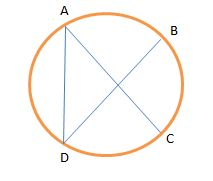In the circle above, the length of arc BC is 100 degrees, and the segment AC is a diameter. What is the measure of angle ADB in degrees?

100

40

90

80

cannot be determined

40

Explanation:

Since we know that segment AC is a diameter, this means that the length of the arc ABC must be 180 degrees. This means that the length of the arc AB must be 80 degrees.

Since angle ADB is an inscribed angle, its measure is equal to half of the measure of the angle of the arc that it intercepts. This means that the measure of the angle is half of 80 degrees, or 40 degrees.

### Example Question #23 : Circles

What is the angle of a sector of area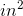on a circle having a radius of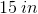?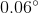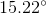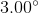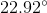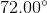Explanation:

To begin, you should compute the complete area of the circle: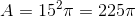Now, to find the angle measure of a sector, you find what portion of the circle the sector is. Here, it is: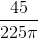Now, multiply this by the total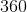degrees in a circle: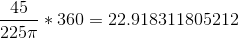Rounded, this is.

### Example Question #11 : Sectors

What is the angle of a sector that has an arc length of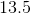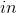on a circle of diameter?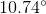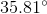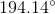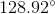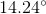Explanation:

The first thing to do for this problem is to compute the total circumference of the circle. Notice that you were given the diameter. The proper equation is therefore: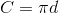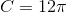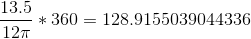Rounded to the nearest hundredth, this is.